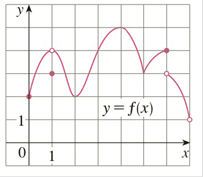Chapter 3.1, Problem 5E

Chapter
Section
Textbook Problem

# Use the graph to state the absolute and local maximum and minimum values of the function.To determine

To state:

The absolute and local maximum and minimum values of the function by using the given graph

Explanation

1) Concept:

Use definitions, extreme value theorem, and the given graph to find whether the given number has an absolute maximum or minimum, a local maximum or minimum, or neither a maximum nor a minimum.

2) Definitions:

i. Let c be a number in the domain D of a function f; then f(c) is the absolute maximum value of f on D if fcf(x) for all x in D.

ii. Let c be a number in the domain D of a function f; then f(c) is the absolute minimum value of f on D if fcf(x) for all x in D.

iii. The number fc is a local maximum value of f if fcf(x) when x is near c.

iv. The number fc is a local minimum value of f if fcf(x) when x is near c.

The extreme value theorem:

If f is continuous on a closed interval a, b, then f attains an absolute maximum value fc and an absolute minimum value fd at some numbers c and d in a, b.

3) Calculation:

At x=4, the function has the greatest y value, so the absolute maximum occurs at the point (4, 5) and absolute maximum value is f4= 5.

At x=7, the function has the smallest y value, but there is a hole at point (7, 1). So this point doesn’t lie in the domain, and there are many points close to 7, but by using extreme value theorem, we cannot say which point is the absolute minimum.

Hence, there is no absolute minimum.

Consider the values of x near x=1, f1fx for all x near x= 1.

Thus, f (1)=3 is a local minimum value.

Now consider the values of x near x=2, so in the interval (1, 4), f2fx for all x near x= 2

### Still sussing out bartleby?

Check out a sample textbook solution.

See a sample solution

#### The Solution to Your Study Problems

Bartleby provides explanations to thousands of textbook problems written by our experts, many with advanced degrees!

Get Started

#### Find f. f(x) = 2 + 12x 12x2, f(0) = 4, f(0) = 12

Single Variable Calculus: Early Transcendentals, Volume I

#### Solve the equations in Exercises 126. x1x=0

Finite Mathematics and Applied Calculus (MindTap Course List)

#### In Exercises 7-28, perform the indicated operations and simplify each expression. 18. 1x+1y11xy

Applied Calculus for the Managerial, Life, and Social Sciences: A Brief Approach

#### Evaluate the definite integral. 12xx1dx

Single Variable Calculus: Early Transcendentals

#### Sometimes, Always, or Never: If 0 ≤ an ≤ bn for all n and diverges, then converges.

Study Guide for Stewart's Single Variable Calculus: Early Transcendentals, 8th